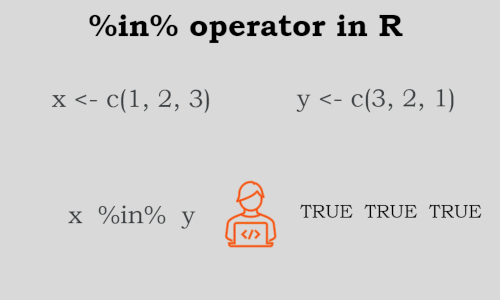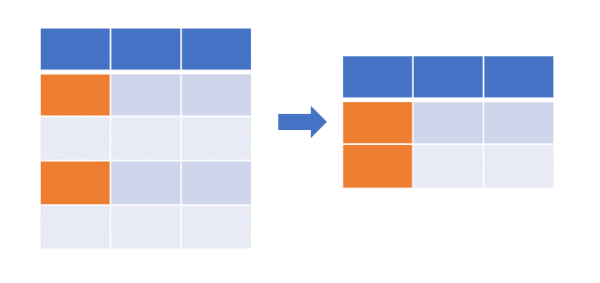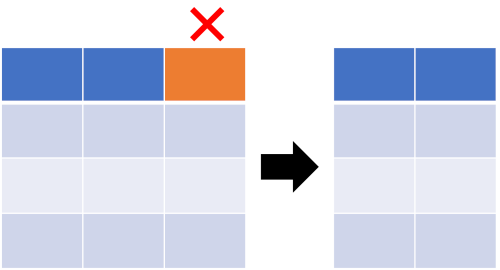# How to use `%in%` and `%notin%` operators in R (with examples)### Introduction

• `%in%` is a built-in infix operator, which is similar to value matching function `match`. `%in%` is an infix version of `match`. User defined infix operators can be created by creating a function and naming it in between two `%` (e.g. `%function_name%`).
• `%in%` returns logical vector (TRUE or FALSE but never NA) if there is a match or not for its left operand. Output logical vector has the same length as left operand.
• If there are two vector `x` and `y` then the syntax of `%in%`: `x %in% y`
• `%in%` works only with vectors
• `%notin%` is not a built-in operator and can be created by negating the `%in%` operator (see below)
• Help syntax for `%in%` operator: `?"%in%"`

Learn about `\$` operator for data extraction from Data Frame and list in R

Here are few examples of how to use `%in%` to manipulate vectors and Data Frames in R,

### `%in%` to check the value in a vector

`%in%` is helpful to check any value in a vector. If there is a match to the value, it returns TRUE, otherwise FALSE

``````x <- c(1,5,10,20,20,24,45)

# check any number in x vector
20 %in% x
 TRUE
``````

### `%in%` to compare two vectors

`%in%` operator is useful to compare two vectors and identify common values in between the two vectors.

``````x <- c(1,5,45)
y <- c(5,45)

x %in% y
 FALSE  TRUE  TRUE

# find common values
x[x %in% y]
  5 45
``````

Check two sequences of numbers and identify common numbers

``````x <- 1:5
y <- 3:7

x %in% y
 FALSE FALSE  TRUE  TRUE  TRUE

# find common letters
x[x %in% y]
 3 4 5
``````

Similarly, you can check two vectors containing letters and identify common letters

``````x <- LETTERS[1:5]
y <- LETTERS[4:7]

x %in% y
 FALSE FALSE FALSE  TRUE  TRUE

# find common numbers
x[x %in% y]
 "D" "E"
``````

If you have a big vector (say vector with 1000 values), you can use `any`, `all`, or `which` functions with `%in%` operator

``````x <- 1:1000
y <- 900:2000

# check if there is any common values between a and b vectors
any(x %in% y)
 TRUE

# check if there are all values common between a and b vectors
all(x %in% y)
 FALSE

# get indexes of common values
a <- 1:10
b <- 6:200

which(a %in% b)
  6  7  8  9 10
``````

### `%in%` to check the value in a Data Frames

`%in%` can be used for checking any value present in columns of Data Frames

Create a Data Frame,

``````df <- data.frame(col1 = c("A", "B", "C"),
col2 = c(1, 2, 3),
col3 = c(0.1, 0.2, 0.3))
# output
col1 col2 col3
1    A    1  0.1
2    B    2  0.2
3    C    3  0.3
``````

Check if any value is present in Data Frame columns,

``````# check if 'B' is present in col1
'B' %in% df\$col1
 TRUE

# to check if any value in col1  is B
df\$col1  %in% 'B'
 FALSE  TRUE FALSE
``````

If you want to compare the vector with Data Frame columns, the `%in%` operator comes in handy. See below example,

``````# check if values of vector are present in a Data Frame columns
lapply(df, `%in%`, c(1, 4, 0.1))
# output
\$col1
 FALSE FALSE FALSE

\$col2
  TRUE FALSE FALSE

\$col3
  TRUE FALSE FALSE
``````

### `%in%` to update the values in a Data Frame

`%in%` operator is useful when you want to scan Data Frame and update (replace) the existing values in Data Frame where there is a match with a given vector or values.

``````# search values in vector in Data Frame and replace with 0 where there is match
df[sapply(df, `%in%`, c(1, 4, 0.1))] <- 0
df
# output
col1 col2 col3
1    A    0  0.0
2    B    2  0.2
3    C    3  0.3
``````

### `%in%` and `%notin%` to filter (subset) Data Frames based on multiple values (with dplyr)In filtering the Data Frame based on single or multiple values, the `%in%` operator is useful. You can use the `%in%` operator to filter the values in columns in Data Frame,

Filter (subset) Data Frame where multiple values from vector match to the values in `col1`,

``````library(dplyr)
df  %>% filter(col1 %in% c('A', 'B'))  # same as df[df\$col1 %in% c('A', 'B'),]
# output
col1 col2 col3
1    A    1  0.1
2    B    2  0.2
``````

Filter (subset) Data Frame where multiple values does not match to the values in `col1` using `%notin%`,

For this example, you need to first create `%notin%` operator (see below at the end of this article)

``````library(dplyr)
df  %>% filter(col1 %notin% 'C')
# output
col1 col2 col3
1    A    1  0.1
2    B    2  0.2
``````

### `%in%` and `%notin%` to remove column from Data Frames`%in%` and `%notin%` operators can also be used for removing single or multiple columns from Data Frames

You need to first create the `%notin%` operator (see below at the end of this article)

``````# remove col2
df[ , !(names(df) %in% "col2")]
# output
col1 col3
1    A  0.1
2    B  0.2
3    C  0.3

#  %notin% operator to remove columns.
#  You need to first create %notin% operator (see below at the end of this article)
df[ , (names(df) %notin% "col2")]
# output
col1 col3
1    A  0.1
2    B  0.2
3    C  0.3

# to remove multiple column use column names vector such as c("col2", "col3")
``````

### `%in%` to select columns from Data Frames

`%in%` can be used to select single or multiple columns from Data Frames

``````# select single column
df[ ,(names(df) %in% "col3"), drop=FALSE]
# output
col3
1  0.1
2  0.2
3  0.3

# select multiple columns
df[ ,(names(df) %in% c("col1", "col2"))]
# output
col1 col2
1    A    1
2    B    2
3    C    3
``````

`%in%` can also be used for selecting specific columns where the column names match on a condition,

Select the columns from dataframe where column names matches row names of other dataframe

``````# create dataframe with rownames
df_row <- data.frame(M1 = c("X", "Y" ),
M2 = c(11, 22), row.names = c("col1", "col3"))

# get columns of dataframe df where its column names matches
# to rownames of dataframe df_row
df[,  names(df) %in% rownames(df_row)]
# output
col1 col3
1    A  0.1
2    B  0.2
3    C  0.3
``````

### `%in%` to compare two Data Frames

`%in%` can be used to compare two Data Frames and subset Data Frames based on the column value match. This is more similar like left join query i.e. select all records from one Data Frame where column values match to another Data Frame

Create another Data Frame,

``````df2 <- data.frame(col1 = c("A", "B", "D", "E"),
col4 = c(100, 200, 300, 400),
col5 = c("a", "b", "c", "d"))

df2
# output
col1 col4 col5
1    A  100    a
2    B  200    b
3    D  300    c
4    E  400    d
``````

Now compare `df` and `df2` to get all records from `df2` where `col1` values match to `col1` values in df (similar to left join of tables),

``````subset(df2, df2\$col1 %in% df\$col1)
# output
col1 col4 col5
1    A  100    a
2    B  200    b
``````

### Comparison of `%in%` and `==` operators

• `==` operator compares the value between two vectors element-wise (the first value of one vector compared with the first value of another vector), whereas `%in%` compares the value between two vectors one by all (the first value of the first vector compared with all values of the second vector)
• With `==` operator, the length of the left and right operands must be the same. It is not necessary to have the same length for left and right operands for `%in%` operator.
``````x <- c(1, 2, 3)
y <- c(3, 2, 1)

x %in% y
 TRUE TRUE TRUE

x == y
 FALSE  TRUE FALSE

# compare and get indexes of two vectors
a <- c(1, 2, 9, 2)
b <- c(1, 2, 3, 4, 5)

# == operator only found first two indexes
which(a == b)
 1 2
Warning message:
In a == b : longer object length is not a multiple of shorter object length

# %in% operator found all matched value indexes
which(a %in% b)
 1 2 4
``````

### Create `%notin%` operator (opposite to `%in%`)

`%notin%` operator is not built-in and can be created by applying `Negate` function to `%in%`.

`%notin%` is opposite to `%in%` operator

You can also use `%notin%` as by putting `!` in front of the `%in%` expression (`!%in%`)

```````%notin%` <- Negate(`%in%`)
``````

Check the value in a vector using `%notin%`

``````x <- c(1,5,10,20,20,24,45)

# check any number in x vector
50 %notin%  x # same as !(50 %in%  x)
 TRUE
``````

Update values of Data Frame to NA where values do not match,

``````# create data frame
df <- data.frame(col1 = c("A", "B", "C"),
col2 = c(1, 2, 3),
col3 = c(0.1, 0.2, 0.3))

# update values of Data Frame to NA where values does not match to c(2, 3)
df[sapply(df, `%notin%`, c(2, 3))] <- NA  # df[sapply(!(df, `%in%`, c(2, 3)))] <- NA
df
# output
col1 col2 col3
1 <NA>   NA   NA
2 <NA>    2   NA
3 <NA>    3   NA
``````

## Enhance your skills with courses R

If you have any questions, comments or recommendations, please email me at reneshbe@gmail.com